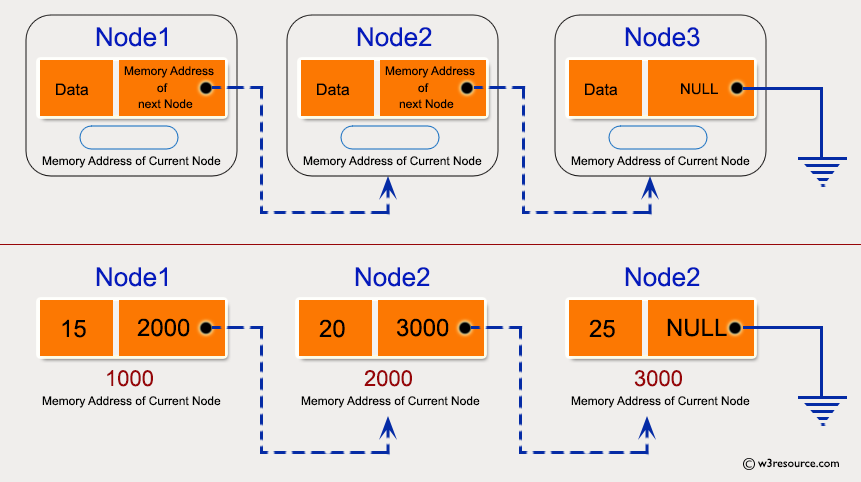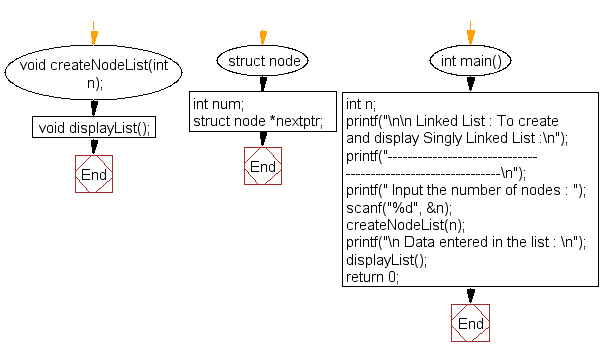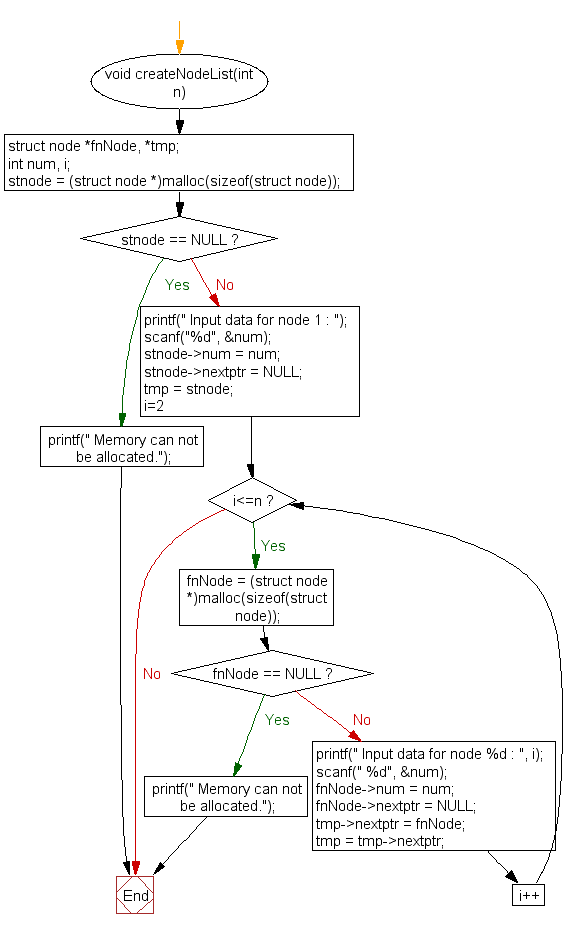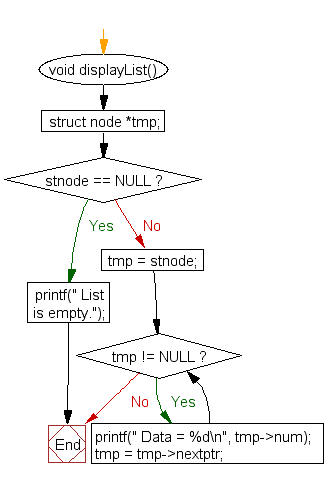﻿ C exercises: To create and display Singly Linked List - w3resource# C Exercises: To create and display Singly Linked List

## C Linked List : Exercise-1 with Solution

Write a program in C to create and display Singly Linked List.

Pictorial Presentation:Sample Solution:

C Code:

``````#include <stdio.h>
#include <stdlib.h>

struct node
{
int num;                        //Data of the node
struct node *nextptr;           //Address of the next node
}*stnode;

void createNodeList(int n); // function to create the list
void displayList();         // function to display the list

int main()
{
int n;
printf("\n\n Linked List : To create and display Singly Linked List :\n");
printf("-------------------------------------------------------------\n");

printf(" Input the number of nodes : ");
scanf("%d", &n);
createNodeList(n);
printf("\n Data entered in the list : \n");
displayList();
return 0;
}
void createNodeList(int n)
{
struct node *fnNode, *tmp;
int num, i;
stnode = (struct node *)malloc(sizeof(struct node));

if(stnode == NULL) //check whether the fnnode is NULL and if so no memory allocation
{
printf(" Memory can not be allocated.");
}
else
{
// reads data for the node through keyboard

printf(" Input data for node 1 : ");
scanf("%d", &num);
stnode->num = num;
tmp = stnode;
for(i=2; i<=n; i++)
{
fnNode = (struct node *)malloc(sizeof(struct node));
if(fnNode == NULL)
{
printf(" Memory can not be allocated.");
break;
}
else
{
printf(" Input data for node %d : ", i);
scanf(" %d", &num);

fnNode->num = num;      // links the num field of fnNode with num
fnNode->nextptr = NULL; // links the address field of fnNode with NULL

tmp->nextptr = fnNode; // links previous node i.e. tmp to the fnNode
tmp = tmp->nextptr;
}
}
}
}
void displayList()
{
struct node *tmp;
if(stnode == NULL)
{
printf(" List is empty.");
}
else
{
tmp = stnode;
while(tmp != NULL)
{
printf(" Data = %d\n", tmp->num);       // prints the data of current node
tmp = tmp->nextptr;                     // advances the position of current node
}
}
}
```
```

Sample Output:

``` Linked List : To create and display Singly Linked List :
-------------------------------------------------------------
Input the number of nodes : 3
Input data for node 1 : 5
Input data for node 2 : 6
Input data for node 3 : 7

Data entered in the list :
Data = 5
Data = 6
Data = 7
```

Flowchart:createNodeList() :displayList() :C Programming Code Editor:

Have another way to solve this solution? Contribute your code (and comments) through Disqus.

What is the difficulty level of this exercise?

﻿